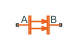# Radiative Heat Transfer

Heat transfer by radiation

• Library:
• Simscape / Foundation Library / Thermal / Thermal Elements

•## Description

The Radiative Heat Transfer block represents a heat transfer by radiation between two bodies. The transfer is governed by the Stefan-Boltzmann law:

`$Q=k\cdot A\cdot \left({T}_{A}^{4}-{T}_{B}^{4}\right),$`

where:

• Q is heat flow.

• k is radiation coefficient.

• A is emitting body surface area.

• D is distance between layers (that is, thickness of material).

• TA and TB are temperatures of the two bodies.

The radiation coefficient is determined by geometrical shapes, dimensions, and surface emissivity. For example, the radiation constant for the heat transfer between two parallel plates is computed as

`$k=\frac{\sigma }{\frac{1}{{\epsilon }_{1}}+\frac{1}{{\epsilon }_{2}}-1},$`

where:

• σ is Stefan-Boltzmann constant.

• ε1 is surface emissivity for the emitting plate.

• ε2 is surface emissivity for the receiving plate.

Similarly, the radiation coefficient for concentric cylinders is determined with the formula

`$k=\frac{\sigma }{\frac{1}{{\epsilon }_{1}}+\frac{1-{\epsilon }_{2}}{{\epsilon }_{2}}\frac{{r}_{1}}{{r}_{2}}},$`

where r1 and r 2 are the emitting and receiving cylinder radii, respectively. For more information, including formulas for a wide variety of shapes, see .

Connections A and B are thermal conserving ports associated with the emitting and receiving bodies, respectively. The block positive direction is from port A to port B. This means that the heat flow is positive if it flows from A to B.

### Variables

To set the priority and initial target values for the block variables prior to simulation, use the Initial Targets section in the block dialog box or Property Inspector. For more information, see Set Priority and Initial Target for Block Variables.

Nominal values provide a way to specify the expected magnitude of a variable in a model. Using system scaling based on nominal values increases the simulation robustness. Nominal values can come from different sources, one of which is the Nominal Values section in the block dialog box or Property Inspector. For more information, see Modify Nominal Values for a Block Variable.

## Ports

### Conserving

expand all

Thermal conserving port associated with body A.

Thermal conserving port associated with body B.

## Parameters

expand all

Radiating body area of heat transfer.

Radiation coefficient of the two bodies, based on their geometrical shapes, dimensions, and surface emissivity. For more information, see .

 Siegel, R. and J.R. Howell. Thermal Radiation Heat Transfer. New York: Taylor and Francis, 2002.